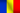﻿ Research Laboratory## Research Directions

• PDE. Solution analysis for classes of elliptic problems. The study of locating and multiplicating radial solutions of stationary,elliptical systems with different behaviors
• Study the development of models based on classes of functions defined over elliptic curves space. Study at the boundary of non-supersingular elliptic equations models. Applications in cryptography
• Developing classes of finite sets parameters of eigenfunctions in which a particular set of values that has properties at the boundary in the sense of reseting points for the next levels of fractals
• Study the development of mathematical models applicable to information structures, based on homogeneous operators
• Mathematical models in economics - finance, banking, structural modeling